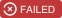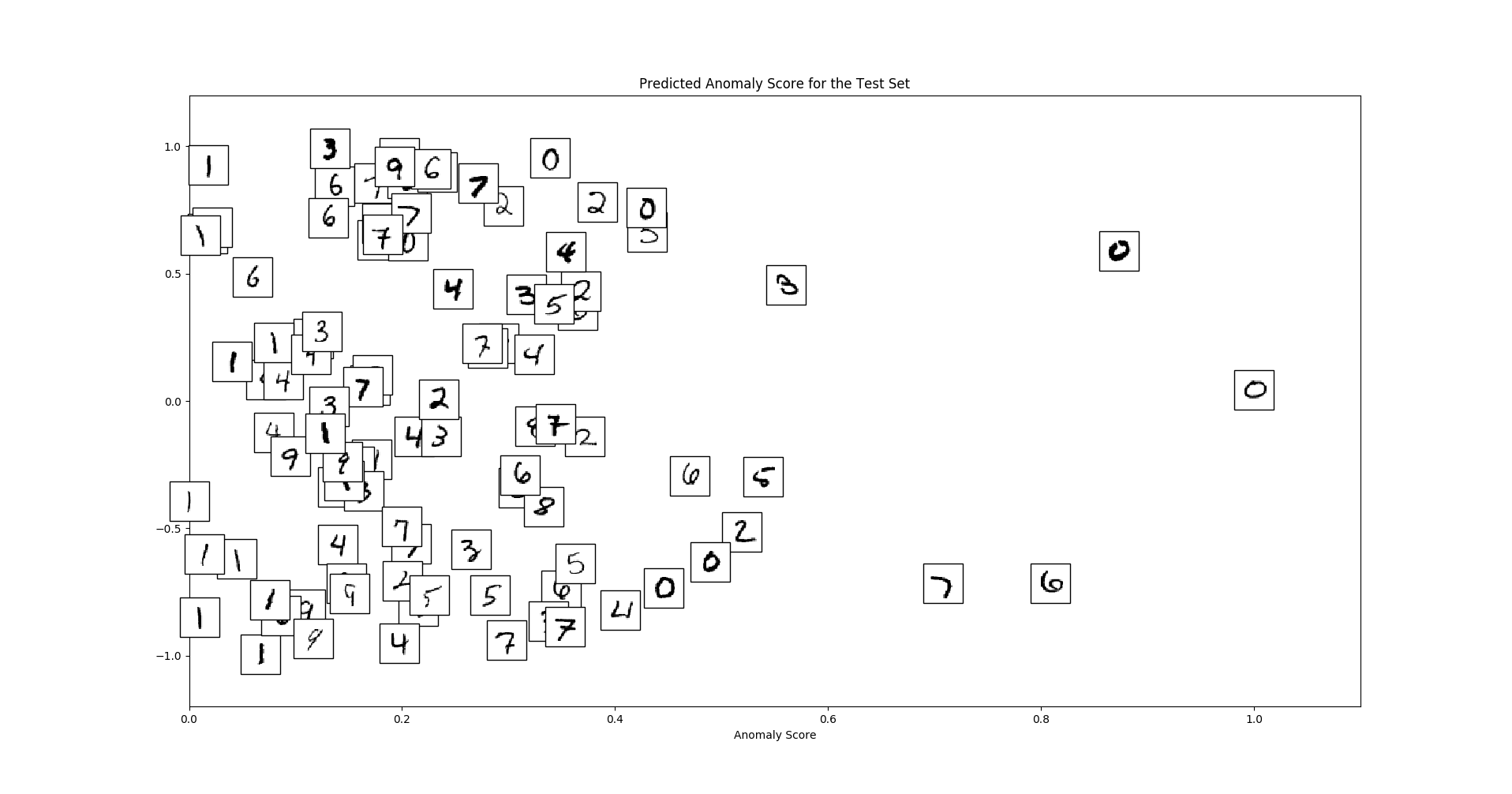Click the “chat” button below for chat support from the developer who created it, or find similar developers for support.KDD-OpenSource402 Stars89 ForksMIT License135 Commits9 Opened issues#### Description

Repository of the paper "A Systematic Evaluation of Deep Anomaly Detection Methods for Time Series".!
?

#### Need anything else?# Anomaly Detection on Time Series: An Evaluation of Deep Learning Methods.The goal of this repository is to provide a benchmarking pipeline for anomaly detection on time series data for multiple state-of-the-art deep learning methods.

## Implemented Algorithms

| Name | Paper | |--------------------|---------------------| | LSTM-AD | Long short term memory networks for anomaly detection in time series, ESANN 2015 | | LSTM-ED |LSTM-based encoder-decoder for multi-sensor anomaly detection, ICML 2016| | Autoencoder | Outlier detection using replicator neural networks, DaWaK 2002 | | Donut| Unsupervised Anomaly Detection via Variational Auto-Encoder for Seasonal KPIs in Web Applications, WWW 2018 | | REBM | Deep structured energy based models for anomaly detection, ICML 2016| |DAGMM| Deep autoencoding gaussian mixture model for unsupervised anomaly detection, ICLR 2018| |LSTM-DAGMM | Extension of DAGMM using an LSTM-Autoencoder instead of a Neural Network Autoencoder|

## Usage

```git clone git://github.com/KDD-OpenSource/DeepADoTS.git
virtualenv venv -p /usr/bin/python3
source venv/bin/activate
pip install -r requirements.txt
python3 main.py
```

## Example

We follow the scikit-learn API by offering the interface methods

`fit(X)`
and
`predict(X)`
. The former estimates the data distribution in an unsupervised way while the latter returns an anomaly score for each instance - the higher, the more certain is the model that the instance is an anomaly. To compare the performance of methods, we use the ROC AUC value.

We use MNIST to demonstrate the usage of a model since it is already available in TensorFlow and does not require downloading external data (even though the data has no temporal aspect).

```import pandas as pd
import tensorflow as tf
from sklearn.metrics import roc_auc_score

from src.algorithms import AutoEncoder
from src.datasets import Dataset
class MNIST(Dataset):
"""0 is the outlier class. The training set is free of outliers."""
```def __init__(self, seed):
super().__init__(name="MNIST", file_name='')  # We do not need to load data from a file
self.seed = seed

# 0 is the outlier, all other digits are normal
OUTLIER_CLASS = 0
mnist = tf.keras.datasets.mnist
(x_train, y_train), (x_test, y_test) = mnist.load_data()
# Label outliers with 1 and normal digits with 0
y_train, y_test = (y_train == OUTLIER_CLASS), (y_test == OUTLIER_CLASS)
x_train = x_train[~y_train]  # Remove outliers from the training set
x_train, x_test = x_train / 255, x_test / 255
x_train, x_test = x_train.reshape(-1, 784), x_test.reshape(-1, 784)
self._data = tuple(pd.DataFrame(data=data) for data in [x_train, y_train, x_test, y_test])```
x_train, y_train, x_test, y_test = MNIST(seed=0).data()
Use fewer instances for demonstration purposes
x_train, y_train = x_train[:1000], y_train[:1000]
x_test, y_test = x_test[:100], y_test[:100]
model = AutoEncoder(sequence_length=1, num_epochs=40, hidden_size=10, lr=1e-4)
model.fit(x_train)
error = model.predict(x_test)
print(roc_auc_score(y_test, error))  # e.g. 0.8614
```

We can visualize the samples with respective error values as follows ```python import numpy as np import matplotlib.pyplot as plt from matplotlib import offsetbox

"""Borrowed from https://github.com/scikit-learn/scikit-learn/blob/master/examples/manifold/plotlledigits.py#L44""" error = (error - error.min()) / (error.max() - error.min()) # Normalize error xtest = xtest.values yrandom = np.random.rand(len(xtest)) * 2 - 1 plt.figure(figsize=(20, 10)) ax = plt.subplot(111) if hasattr(offsetbox, 'AnnotationBbox'): shownimages = np.array([[1., 1.]]) for i in range(len(xtest)): Xinstance = [error[i], yrandom[i]] dist = np.sum((Xinstance - shownimages) ** 2, 1) if np.min(dist) < 4e-5: # don't show points that are too close continue shownimages = np.r[shownimages, [Xinstance]] imagebox = offsetbox.AnnotationBbox(offsetbox.OffsetImage(xtest[i].reshape(28, 28), cmap=plt.cm.grayr), Xinstance) ax.addartist(imagebox) plt.xlim((0, 1.1)) plt.ylim((-1.2, 1.2)) plt.xlabel("Anomaly Score") plt.title("Predicted Anomaly Score for the Test Set") plt.show() ``` Which creates a plot like thisWe can see that global outliers (zeros) and local outliers (strangely written digits) receive high anomaly scores.

## Deployment

• `docker build -t deep-adots .`
• `docker run -ti deep-adots /bin/bash -c "python3.6 /repo/main.py"`

## Authors/Contributors

Supervisors: * Lukas Ruff * Fabian Geier * Emmanuel Müller